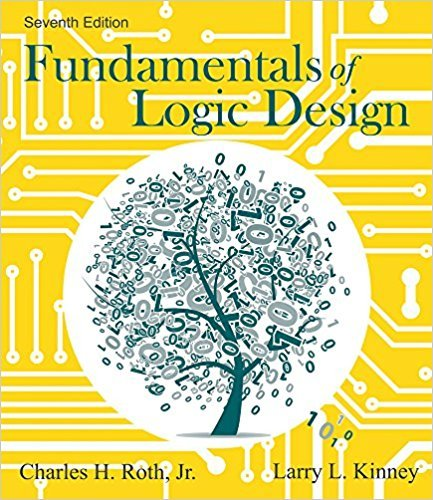×
Log in to StudySoup
Get Full Access to Fundamentals Of Logic Design - 7 Edition - Chapter 1 - Problem 1.1
Join StudySoup for FREE
Get Full Access to Fundamentals Of Logic Design - 7 Edition - Chapter 1 - Problem 1.1

Already have an account? Login here
×
Reset your password

# Convert to hexadecimal and then to binary: (a) 757.2510ISBN: 9781133628477 165

## Solution for problem 1.1 Chapter 1

Fundamentals of Logic Design | 7th Edition

• Textbook Solutions
• 2901 Step-by-step solutions solved by professors and subject experts
• Get 24/7 help from StudySoup virtual teaching assistantsFundamentals of Logic Design | 7th Edition

4 5 1 304 Reviews
22
3
Problem 1.1

Convert to hexadecimal and then to binary: (a) 757.2510 (b) 123.1710 (c) 356.8910 (d) 1063.510

Step-by-Step Solution:

Q:Convert to hexadecimal and then to binary:(a) 757.25 (b) 123.17 (c) 356.89 (d) 1063.5 Step By Step SolutionStep 1 of 4:(a)Converting 757.25 to hexadecimal and then to Binary:Hexadecimal:1.1 Dividing 757 by 16: Quotient:47, reminder:5 Dividing 47 by 16:Quotient 2, reminder:15=F 16 Dividing 2 by 16:Quotient:0, reminder 2 Fraction part: Multiplying 0.25 by 16=4 Therefore Hexadecimal is (2F5.4) 16 1.2: Converting #2F5.4 to Binary=(10111101.010) 2Therefore:

Step 2 of 1

##### ISBN: 9781133628477

This textbook survival guide was created for the textbook: Fundamentals of Logic Design, edition: 7. Fundamentals of Logic Design was written by and is associated to the ISBN: 9781133628477. The full step-by-step solution to problem: 1.1 from chapter: 1 was answered by , our top Engineering and Tech solution expert on 11/23/17, 05:09AM. Since the solution to 1.1 from 1 chapter was answered, more than 1089 students have viewed the full step-by-step answer. The answer to “Convert to hexadecimal and then to binary: (a) 757.2510 (b) 123.1710 (c) 356.8910 (d) 1063.510” is broken down into a number of easy to follow steps, and 15 words. This full solution covers the following key subjects: . This expansive textbook survival guide covers 20 chapters, and 727 solutions.

Unlock Textbook Solution

Enter your email below to unlock your verified solution to:

Convert to hexadecimal and then to binary: (a) 757.2510